You are here: Start » AVL.NET » Image Combinators

# Image Combinators

IconNameDescription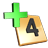AddImagesAdds two images pixel by pixel.AddImages_OfArrayAdds images of an array pixel by pixel.AddImages_OfLoopAdds images appearing in consecutive iterations pixel by pixel.AverageImagesAverages two images pixel by pixel.AverageImages_OfArrayAverages images from an array pixel by pixel.BlendImagesComputes weighted sum pixel by pixel.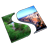ComposeImagesTakes pixels from the first image within the specified region and from the other one elsewhere.DifferenceImageComputes the non-negative distances between corresponding pixel values.DifferenceImage_FlexComputes the non-negative distances between corresponding pixel values using tiles.DifferenceImage_ShiftedComputes the non-negative distances between corresponding pixel values.DivideImagesDivides two images pixel by pixel.LerpImagesInterpolates two images linearly pixel by pixel.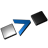MaximumImageCreates an image from the higher pixel values of each corresponding pair.MaximumImage_OfArrayComputes the maximum of images of an array pixel by pixel.MaximumImage_OfLoopComputes the maximum of images appearing in consecutive iterations pixel by pixel.MedianImages_OfArrayCompute median value for each pixel of images from an array.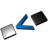MinimumImageCreates an image from the lower pixel values of each corresponding pair.MinimumImage_OfArrayComputes the minimum of images of an array pixel by pixel.MinimumImage_OfLoopComputes the minimum of images appearing in consecutive iterations pixel by pixel.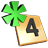MultiplyImagesMultiplies two images pixel by pixel.MultiplyImages_OfArrayMultiplies images of an array pixel by pixel.MultiplyImages_OfLoopMultiplies images appearing in consecutive iterations pixel by pixel.NthImage_OfArrayFor each pixel location, get value of n-th (increasing) pixel among input images.SubtractImagesSubtracts two images pixel by pixel. The result is signed.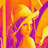VisualizeHeatMapColorizes heat-map and blends it with background image